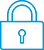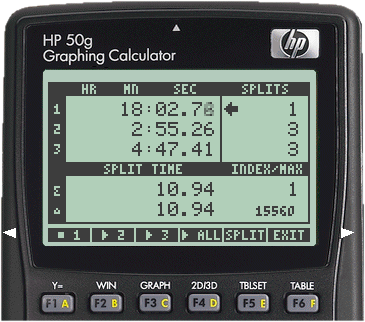cancel
Showing results for
Search instead for
Did you mean:It has been a while since anyone has replied. Simply ask a new question if you would like to start the discussion again.Level 1
5 2 0 1
Message 1 of 5
919
Flag Post

# Present value of a bondHP Recommended
HP 10bII+ Financial Calculator

I am trying to solve the following problem

Bond P is a premium bond with a coupon rate of 9 percent. Bond D is a discount bond with a 5 percent coupon rate. Both bonds make semiannual payments, have a yield to maturity of 7 percent, and have five years to maturity. What is the price for bond P? For bond D? What is the duration of bond P? For bond D? (Assume a \$1000 face value, settlement date of 1 January 2010 and a maturity date of 31 December 2014).

My inputs so far are

01.012010 SetDate

31.122014 MatDate

9 CPN%

7 YTM

When I hit the PRICE key I get 108.3123. In excel the answer is 1,083.17 and I'm pretty sure I'm not getting this in the calculator because I'm not inputting the face value of 1,000 so the calculator uses its default value of 100; problem is I have not been able to figure out how to add the face value, and no amount of user guide, youtube tutorial, and forums has helpmed me with this.

The date setting on the calculator is D.MY and the payment is set to SEMI

Any help is greatly appreciated.

Tags (1)
4 REPLIES 4Level 6
140 139 29 60
Message 2 of 5
Flag PostHP Recommended

PRICE on the 10bII+ is defined as "price per 100.00 face value for a given yield".  That being the case, a bond with a face value of 1000 will require you to multiply your price result by 10 to obtain the final answer.

Also, it may simply be a difference in local terminology, but using a MatDate of 31.122014 in this case may be throwing off your calculation slightly from what is expected.  It is one day short of a full 5-year term.  If you use 1.012015 as the MatDate, I think you'll find that the result matches what you see from your Excel calculation.

You could also use the TVM methods to solve this.  I don't have a 10bII to verify this, but I believe the following values would work:

END mode

P/YR: 2 (semiannual)

n: 10 (5 years * 2 pmts/year)

i: 7 (YTM)

PMT: 45 ((1000 * 9%)/2)

FV: 1000 (face value)

Solve for PV: -1083.17

The Bond D calculations would be similar to the above, of course.Level 6
305 297 32 76
Message 3 of 5
Flag PostHP Recommended

Apart from the factor 10, which has been explained by David_M, the result you obtain by pressing PRICE is not the total price or net price you have to pay.

The total price is the sum of PRICE and Accrued interest.

See the example on pages 106-107 of the User Guide.

http://h10032.www1.hp.com/ctg/Manual/c02989763.pdf

Maybe this also explains the slightly different results (apart from the factor 10)  for Excel and the calculator.

It could be that they use different definitions for PRICE and Accrued interest but that their sum is the same, and it is this sum which really matters.Level 6
140 139 29 60
Message 4 of 5
Flag PostHP Recommended

@Jan_D wrote:

Apart from the factor 10, which has been explained by David_M, the result you obtain by pressing PRICE is not the total price or net price you have to pay.

The total price is the sum of PRICE and Accrued interest.

See the example on pages 106-107 of the User Guide.

http://h10032.www1.hp.com/ctg/Manual/c02989763.pdf

Maybe this also explains the slightly different results (apart from the factor 10)  for Excel and the calculator.

It could be that they use different definitions for PRICE and Accrued interest but that their sum is the same, and it is this sum which really matters.

Part of the reason that I mentioned the issue with the maturity date is that the OP originally stated that "in excel the answer is 1,083.17".  I don't know for sure the exact formula they used, but the following gives the same result they indicated (more precisely 1083.166053):

`=PRICE(DATE(2010,1,1),DATE(2015,1,1),0.09,0.07,100,2,0)*10`

(Note that the Excel PRICE function also provides its result per 100.0 face value, so the result here must be multiplied by 10 as well)

Changing the maturity date parameter to DATE(2014,12,31) in an effort to match the original question changes the Excel result to 1083.12.  So we are left to guess at what was really intended here since the reported results don't match the dates given in the problem description.

Wouldn't accrued interest only come into play if the holding period of the bond doesn't match the issue and maturity dates?  Whether that is true isn't clear from the problem description as written.  We also don't know if the basis should be 360 or actual, which could potentially change the results if accrued interest applies.

The more I look at different bond examples, the more I remember how messy they can be.  There are many built-in assumptions in the various problems I've run across, and it's not always clear what those assumptions are when the problems are taken out of context.  Unless the original poster is willing to participate by clarifying the details of their request, I don't see how anyone could provide a definitive answer.Level 6
305 297 32 76
Message 5 of 5
Flag PostHP Recommended

Thank you.

It seems that the 2 different results for PRICE, namely  108.3123 and  108.317 are the results belonging to the different maturity dates (2014,12,31) and (2015,1,1) respectively, both on the calculator and Excel.

I tried to understand how Excel could give another PRICE belonging to maturity date  (2014,12,31) than the calculator, but since that does not seem to be the case, there is as far as I am concerned no problem.

So you can forget what I said about accrued interest.

Nevertheless, since settlement date (2010,1,1) differs 1 day from payment date (12,31) in 2009, the accrued interest is based on 1 day, and because there is a simple linear relationship between the number of days passed  since the last payment date and accrued interest, the accrued interest can be calculated.

It is (1/181)*4.5=0.0249, where 181 is the number of days between date (2009,12,31) and payment date (2010,6,30), and 4.5 is the coupon payment.

Here we used a Actual day count calendar.

This is very probably also the result the calculator gives.

Finally I think Jcarreno is not interested in all these details but probably simply wants to hear from us  that in order to get a correct PRICE for a bond  with a face value which is not 100, he or she has to do the calculation on the calculator, multiply the result by the face value, and divide this by 100!

† The opinions expressed above are the personal opinions of the authors, not of HP. By using this site, you accept the Terms of Use and Rules of Participation